# 3 Line Chart

This chapter describes the line chart, a type of two-axis chart that presents data as a series of points connected by straight lines.

The line chart is often used to illustrate the dynamics of data over a particular interval of time. Figure 3-1 demonstrates a typical line chart with three series of data.

Figure 3-1 Example of a Line Chart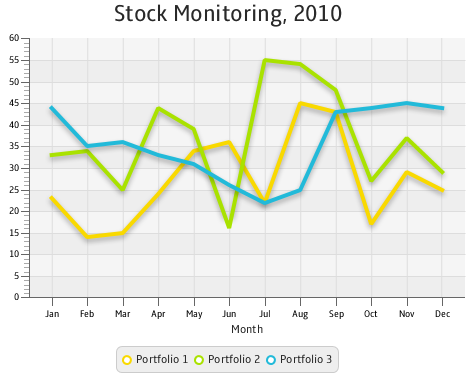Description of "Figure 3-1 Example of a Line Chart"

Each two-axis chart has two axes, the plot of data points, and the legend. You can also specify a title for the chart.

## Creating a Line Chart

To create a line chart, at a minimum, you must define two axes, create the `LineChart` object by instantiating the `LineChart` class, create one or more series of data by using the `XYChart.Series` class, and assign the data to the chart. Example 3-1 implements these tasks.

Example 3-1 Simple Line Chart

```import javafx.application.Application;
import javafx.scene.Scene;
import javafx.scene.chart.LineChart;
import javafx.scene.chart.NumberAxis;
import javafx.scene.chart.XYChart;
import javafx.stage.Stage;

public class LineChartSample extends Application {

@Override public void start(Stage stage) {
stage.setTitle("Line Chart Sample");
//defining the axes
final NumberAxis xAxis = new NumberAxis();
final NumberAxis yAxis = new NumberAxis();
xAxis.setLabel("Number of Month");
//creating the chart
final LineChart<Number,Number> lineChart =
new LineChart<Number,Number>(xAxis,yAxis);

lineChart.setTitle("Stock Monitoring, 2010");
//defining a series
XYChart.Series series = new XYChart.Series();
series.setName("My portfolio");
//populating the series with data

Scene scene  = new Scene(lineChart,800,600);

stage.setScene(scene);
stage.show();
}

public static void main(String[] args) {
launch(args);
}
}
```

In this example, both vertical and horizontal axes are created by using the `NumberAxis` class, a subclass of the `Axis` class, to represent numerical values. Having declared both `X` and `Y` axes numerical, you should specify `Number` parameters for `XYChart.Data` objects when creating a series of data. The first parameters of `XYChart.Data` objects define values for the horizontal axis, whereas, the second parameters of the `XYChart.Data` objects define values for the vertical axis.

The result of compiling and running this application is shown in Figure 3-2.

Figure 3-2 Line Chart with One Series of Data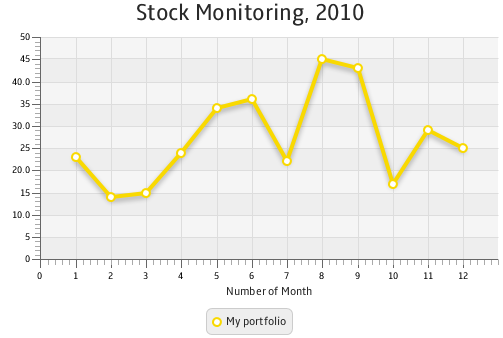Description of "Figure 3-2 Line Chart with One Series of Data"

The line chart shown in Figure 3-2 uses symbols to highlight each data item on the chart. If you want to show trends instead of specific data values on your line chart, you can disable the chart symbols as shown in Example 3-2.

Example 3-2 Disabling Symbols for a Line Chart

```lineChart.setCreateSymbols(false);
```

The sample of a trend chart is shown in Figure 3-1.

In Figure 3-1, axes are shown in their default positions relative to the chart plot. However, you can display an axis on another side of the chart plot by applying the `setSide` method. Example 3-3 demonstrates how to move the horizontal axis to the top of the chart plot.

Example 3-3 Specifying the Axis Side

```xAxis.setSide(Side.TOP);
```

## Creating Categories for a Line Chart

Use the `CategoryAxis` class instead of the `NumberAxis` class to render non-numerical data in a line chart.

Examine the modified code of the application shown in Example 3-4. It creates the horizontal axis by instantiating the `CategoryAxis` class. The declaration of the `LineChart` object is modified to accommodate the change of the X axis type.

Example 3-4 Using Category Axis to Show Months

```import javafx.application.Application;
import javafx.scene.Scene;
import javafx.scene.chart.CategoryAxis;
import javafx.scene.chart.LineChart;
import javafx.scene.chart.NumberAxis;
import javafx.scene.chart.XYChart;
import javafx.stage.Stage;

public class LineChartSample extends Application {

@Override public void start(Stage stage) {
stage.setTitle("Line Chart Sample");
final CategoryAxis xAxis = new CategoryAxis();
final NumberAxis yAxis = new NumberAxis();
xAxis.setLabel("Month");

final LineChart<String,Number> lineChart =
new LineChart<String,Number>(xAxis,yAxis);

lineChart.setTitle("Stock Monitoring, 2010");

XYChart.Series series = new XYChart.Series();
series.setName("My portfolio");

Scene scene  = new Scene(lineChart,800,600);

stage.setScene(scene);
stage.show();
}

public static void main(String[] args) {
launch(args);
}
}
```

The `XYChartData` objects contain the month name and the corresponding numerical value. The label of the horizontal axis is modified accordingly.

The result of compiling and running the modified code of the application is shown in Figure 3-3.

Figure 3-3 Horizontal Category Axis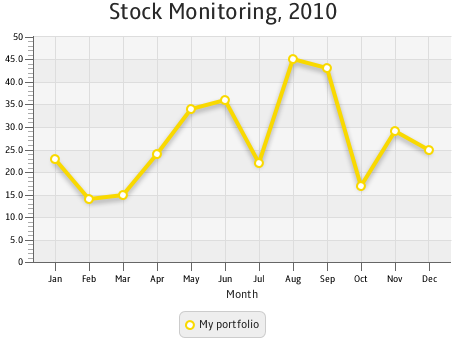Description of "Figure 3-3 Horizontal Category Axis"

Often, line charts enable analyzing different set of data over the same period of time. Use several series of `XYChart.Data` objects to implement this task in your application.

## Adding Series to the Line Chart

Example 3-5 provides source code for the stock monitoring application with three series of data. In addition to the series used in Example 3-4, the previous example, two new series are declared.

The series are assigned to the chart by using consecutive calls of the `getData` and `addAll` methods.

Example 3-5 Adding Two More Series to the Stock Monitoring Sample

```import javafx.application.Application;
import javafx.scene.Scene;
import javafx.scene.chart.CategoryAxis;
import javafx.scene.chart.LineChart;
import javafx.scene.chart.NumberAxis;
import javafx.scene.chart.XYChart;
import javafx.stage.Stage;

public class LineChartSample extends Application {

@Override public void start(Stage stage) {
stage.setTitle("Line Chart Sample");
final CategoryAxis xAxis = new CategoryAxis();
final NumberAxis yAxis = new NumberAxis();
xAxis.setLabel("Month");
final LineChart<String,Number> lineChart =
new LineChart<String,Number>(xAxis,yAxis);

lineChart.setTitle("Stock Monitoring, 2010");

XYChart.Series series1 = new XYChart.Series();
series1.setName("Portfolio 1");

XYChart.Series series2 = new XYChart.Series();
series2.setName("Portfolio 2");

XYChart.Series series3 = new XYChart.Series();
series3.setName("Portfolio 3");

Scene scene  = new Scene(lineChart,800,600);

stage.setScene(scene);
stage.show();
}

public static void main(String[] args) {
launch(args);
}
}
```

Each series of data has its unique name defined by using the `setName` method.

The result of compiling and running this application is shown in Figure 3-4.

Figure 3-4 Stock Monitoring Example with Tree Series of Data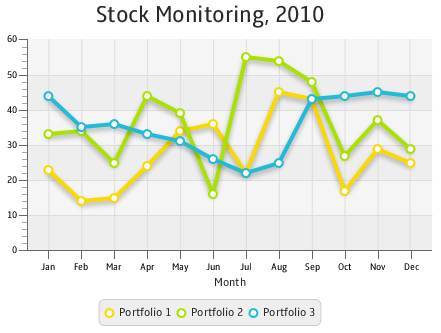Description of "Figure 3-4 Stock Monitoring Example with Tree Series of Data"

Note that the different colors of the lines are defined by the declared order of the corresponding series in the `addAll` method. Change the order as follows: `lineChart.getData().addAll(series3, series1, series2)`, and then compile and run the application. The modified output is shown in Figure 3-5.

Figure 3-5 Alternative Order of Series in the Line Chart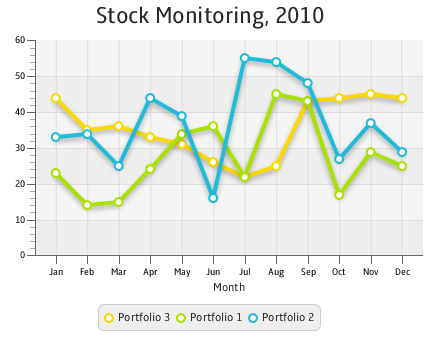Description of "Figure 3-5 Alternative Order of Series in the Line Chart"

Related API Documentation

• `LineChart`

• `Chart`

• `XYChart`

• `XYChart.Data`

• `XYChart.Series`

• `Axis`

• `NumberAxis`

• `CategoryAxis`